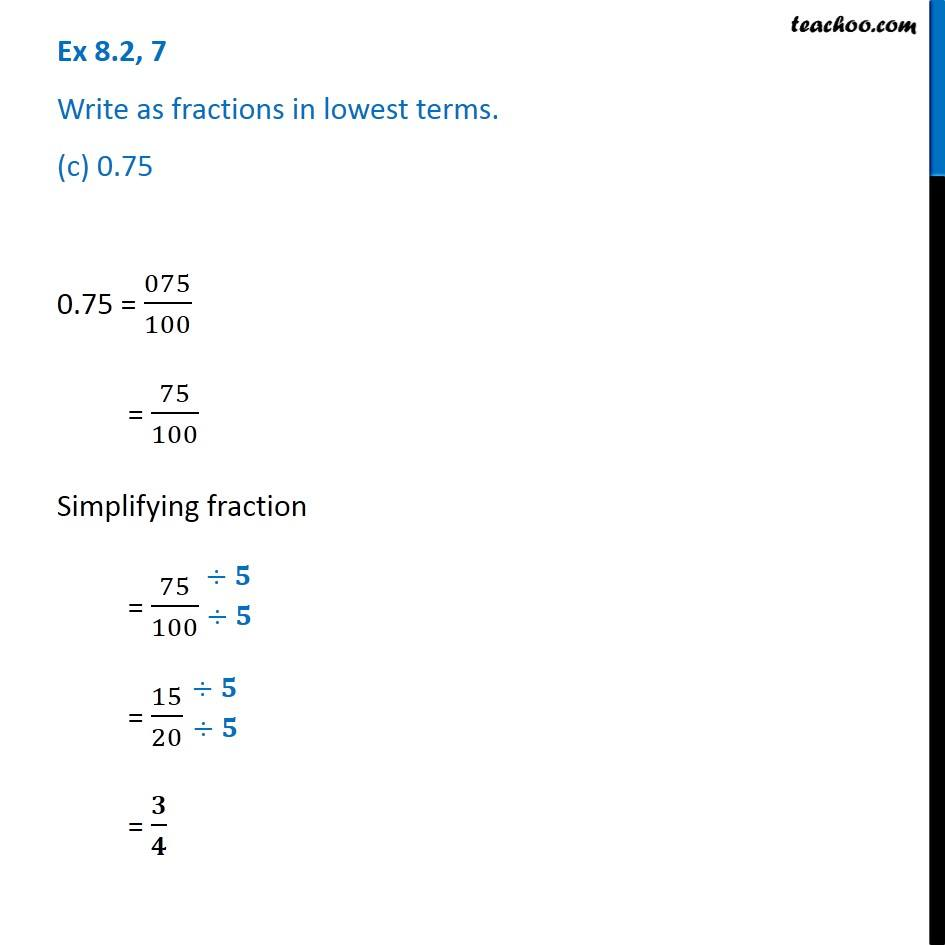1. Chapter 8 Class 6 Decimals
2. Serial order wise
3. Ex 8.2

Transcript

Ex 8.2, 7 Write as fractions in lowest terms. (c) 0.75 0.75 = 075/100 = 75/100 Simplifying fraction = 75/100 = 15/20 = 𝟑/𝟒